Get instant live expert help with Excel or Google Sheets“My Excelchat expert helped me in less than 20 minutes, saving me what would have been 5 hours of work!”

#### Post your problem and you’ll get expert help in seconds.

Your message must be at least 40 characters
Our professional experts are available now. Your privacy is guaranteed.

# How to Use the MATCH Function in Excel

MATCH is a lookup function in Excel that searches for a specified item in a range of cells. It returns the relative position of the item in that range. The range can be a row or column.

## Using the MATCH function in Excel

The Match function can be used to perform an exact lookup, approximate lookup for values that less than or more than the lookup value in a sorted list, and wildcard lookup for any letter in a text lookup value. In this tutorial, we will show you in detail how to use MATCH function with examples.

### Syntax of MATCH

The MATCH function in Excel follows the syntax below:

`=MATCH(lookup_value, lookup_array, [match_type])`

### Arguments

• ##### [match_type]: This value is optional. It is the type of match that the function will perform. The possible values are:

 Match_type Description 1(default) Finds the largest value that is less than or equal to lookup_value. The values in the lookup_array argument must be placed in ascending order. 0 Finds the first value that is exactly equal to lookup_value. The values in the lookup_array argument can be in any order. -1 Finds the smallest value that is greater than or equal tolookup_value. The values in the lookup_array argument must be placed in descending order.

### Returns

The MATCH function returns a numeric value.

If it does not find a match, MATCH will return a #N/A error.

### Note

• The MATCH function is case insensitive. That means it does not distinguish between upper and lowercase when searching for a match.
• MATCH does not return the value of the lookup, it returns the actual position within the lookup_array.
• If the match_type parameter is 0 and the value is a text value, then you can use wildcards in the lookup_value parameter.

 Wild Card Explanation * Matches any sequence of characters ? Matches any single character

You can remove the ‘#N/A’ error returned by the MATCH function if it does not find a match by using the IFERROR function. IFERROR is a conditional function that allows replacing the error itself with a value of your choice. You need to precede the MATCH function with the IFERROR function and decide what value you would like to place in the destination cell to replace the #N/A. Here’s the syntax for IFERROR: `IFERROR(value, value_if_error)`Tips on Managing Errors

### Examples

In the following examples, you will learn how to use the MATCH function in Excel.

###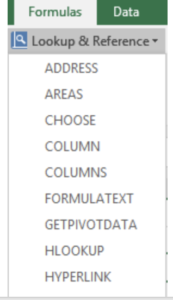Using the MATCH Function Dialog Box

To enter the MATCH function and arguments using the dialog box for the example image:

1. Click on cell E3, which is the location where the result will be displayed.
2. Click on the Formulas tab of the ribbon menu.
3. Choose Lookup and Reference from the ribbon to open the function drop-down list.
4. Click on MATCH in the list to bring up the function’s dialog box.
5. In the dialog box, click on the Lookup_value line.
6. Click on cell E2 in the worksheet to enter the cell reference into the dialog box.
7. Click on the Lookup_array line in the dialog box.
8. Highlight cells A2 to A8 in the worksheet to enter the range in the dialog box.
9. Click on the Match_type line in the dialog box.
10. Enter the number 0 on this line to find an exact match to the data in cell E3.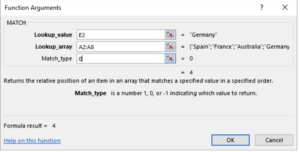11. Click OK to complete the function and close the dialog box.
12. The number 4 appears in cell E3 since the term Germany is the fourth item from the top in the countries list.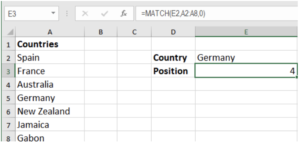When you click on cell E3, the complete function `=MATCH(E2,A2:A8,0) `appears in the formula bar above the worksheet.

### Basic Exact Match

MATCH performs an exact match when match_type is set to 0. The values do not need to be sorted in order for an exact match to work. In the following example, assign the formula` =MATCH(E2,A2:A7,0)` to cell E3 to find the position of Brussels. MATCH here has 0 as match_type and performs an exact match.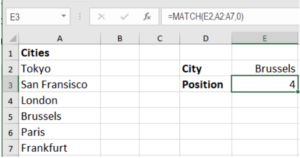### Basic Approximate Match

MATCH performs an approximate match when match_type is set to 1. The values need to be sorted A-Z for this approximate match to work. MATCH will find the largest value equal to or less than the lookup value.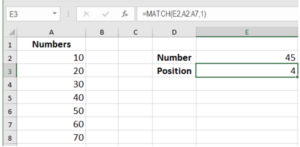In the following example, to find the approximate position of the number 45, assign the formula

`=MATCH(E2,A2:A7,1) `to cell E3. This will return position 4 which is the largest value equal to or less than the lookup value.

### Basic Wildcard Match

When match_type is set to 0, MATCH can perform a match using wildcards. In the example shown below, to find the position of the ID starting with asd*, assign the formula` =MATCH(E2,A2:A7,0) `in cell E3. This will return the position 3 containing the ID asd-43ka.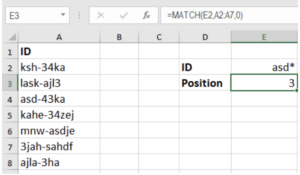### Handling errors with IFERROR

Referring to the previous example, to replace #N/A errors in the MATCH function, assign the formula `=IFERROR(MATCH(E2,A2:A7,0),"Incorrect Lookup Value")` to cell E3.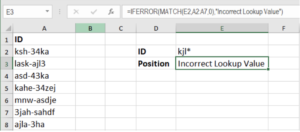Cell E3 now returns Incorrect Lookup Value as the lookup value in cell E2 KJL* does not match with any of the values in column A.

Still need some help with Excel formatting or have other questions about Excel? Connect with a live Excel expert here for some 1 on 1 help. Your first session is always free.

### Did this post not answer your question? Get a solution from connecting with the expert.Another blog reader asked this question today on Excelchat:
Solution examplesI'm having trouble with an index & match formula where I'm looking up two different pieces of info in order to return a specific value.
Solved by K. D. in 54 minsI have two columns with names and a third one with email addresses. For every name that matches, I need to copy the email address to an empty column next to the matching name. .
Solved by E. U. in 15 minsI have 2 sheets. One sheet with the demographics of contractors. eg. Company A, Hispanic, Male. In the second sheet I would like to be able to choose the company from a drop down menu (listing all companies) in column A and have columns B and C automatically populated with Hispanic and Male.
Solved by A. Y. in 34 minsNeed help with a formula, I'd like it to be something similar to '' =IF(AB2=AC2,"Matches","No Match") '' but instead of if they 100% match I want a 5% variable for example, if Cell A1 contains 12:00 and Cell B1 contains 11:59 I want it to class as matching.
Solved by Z. J. in 13 minsI need to find an INDEX function that will convert the Call Day (a number 1-7) to the actual weekday found in row 1 of the DayofWeek named range. I don't know if I'm supposed to use the MATCH function as well or not.
Solved by D. D. in 11 mins## Subscribe to Excelchat.coAnother blog reader asked this question today on Excelchat: